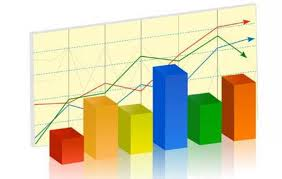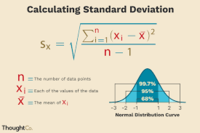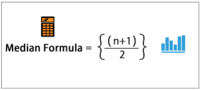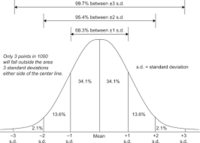STATISTICS

04.05.2020

FLASH DEFINITIONS

What is Statistics?

Statistics are a collection of statistical tools which are used to quantitavely describe or summarize a collection of data.

Descriptive statistics aim to summarize, and as such can be distinguished rom inferential statistics, which are more preictive nature.

Generalizability

Generalizability refers to the ability to draw conclusions about the characteristics of the population as a whole based on the results of data collected from a sample.

This ability is not a give, and depends heavily on the nature of sample collection, sample sixe, and various other factors.

Standard Deviation

The standard deviation of a distribution is the average deviation between individual distribution scores and the distribution's mean.

Individually, the standard deviation provides a good measure of how spread out a disquisitions scores are.

When considered alongside the mean, these two measures provide a good overview of the distribution scores.Skew

When there are more scores toward one end of the distribution than the other, this results in skew.

When the scores of a distribution are more clustered at the high end, the relativel fewer number of scores on the low end result in a tail, with the scenario being referred to as negative skew.

Positive skew is when a distribution shows a tail at its high end.

Variance

Variance is the statistical average of the dispersion of scores in a distribution.

Variance is not often used on its own, but can be useful calculation on the way to a more descriptive statistical measurements, such as standard deviation.Median

The median is a score of a distribution residing at the 50th percentile, separating the top and bottom 50 percent of scores.

The median is useful for both splitting an set distribution scores in half and helping to identify the skew of distribution.Distribution

A distribution is the arrangement of data by the values of one variable in order , from low to high.

This arrangement, and its characteristics, such as shape and spread, provide information about the underlying sample.Source: Data Science App